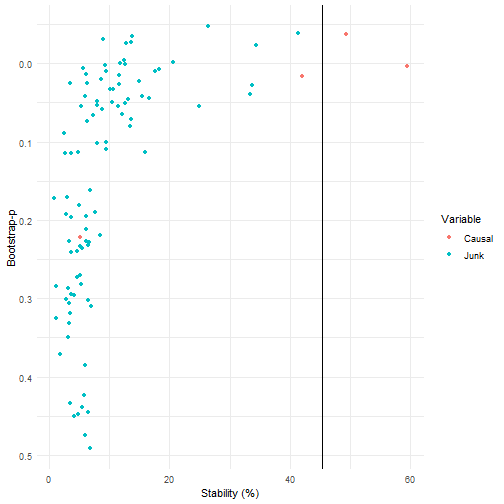The goal of the stabiliser package is to provide a flexible method of applying stability selection (Meinshausen and Buhlmann, 2010) with various model types, and the framework for triangulating the results for multiple models (Lima et al., 2021).

• `stabilise()` performs stability selection on a range of models to identify causal models.
• `triangulate()` identifies which variables are most likely to be causal across all models.
• `stab_plot()` allows visualisation of either `stabilise()` or `triangulate()` outputs.

## Installation

You can install this package from github using the devtools package as follows:

``````install.packages("stabiliser")
``````

Or to get the most recent development version:

``````devtools::install_github("roberthyde/stabiliser")
``````

## Usage

The stabiliser_example dataset is a simulated example with the following properties:

• 1 simulated outcome variable: `y`
• 4 variables simulated to be associated with `y`: `causal1`, `causal2`
• 95 variables simulated to have no association with `y`: `junk1`, `junk2`
``````library(stabiliser)
data("stabiliser_example")
``````

### `stabilise()`

To attempt to identify which variables are truly “causal” in this dataset using a selection stability approach, use the `stabilise()` function as follows:

``````set.seed(8141)
stable_enet <- stabilise(data = stabiliser_example,
outcome = "y")
``````

Access the stability (percentage of 100 bootstrap resamples where a given variable was selected by a given model) results for elastic net as follows:

``````stable_enet\$enet\$stability
#> # A tibble: 100 x 7
#>    variable mean_coefficient ci_lower ci_upper bootstrap_p stability stable
#>    <chr>               <dbl>    <dbl>    <dbl>       <dbl>     <dbl> <chr>
#>  1 causal1              2.82   0.185      6.10           0      85   *
#>  2 causal2              2.17   0.0609     5.31           0      83.5 *
#>  3 causal3              2.82   0.249      6.33           0      71   <NA>
#>  4 junk86               1.51   0.139      4.16           0      69   <NA>
#>  5 junk14               2.71   0.174      6.64           0      65   <NA>
#>  6 junk13               2.14   0.106      5.23           0      63.5 <NA>
#>  7 junk45               2.43   0.127      6.89           0      61.5 <NA>
#>  8 junk88               2.23   0.142      4.78           0      61   <NA>
#>  9 junk90               1.74   0.0429     5.11           0      50.5 <NA>
#> 10 junk26               1.51   0.0417     4.04           0      45.5 <NA>
#> # ... with 90 more rows
``````

This ranks the variables by stability, and displays the mean coefficients, 95% confidence interval and bootstrap p-value. It also displays whether the variable is deemed “stable”, in this case 2 out of the 4 truly causal variables are identified as “stable”, with no false positives.

By default, this implements an elastic net algorithm over 100 bootstrap resamples of the dataset. Stability of each variable is then calculated as the proportion of bootstrap repeats where that variable is selected in the model.

`stabilise()` also permutes the outcome several times (5 by default) and performs the same process on each permuted dataset (20 bootstrap resamples for each by default).

This allows a permutation threshold to be calculated. Variables with a non-permuted stability % above this threshold are deemed “stable” as they were selected in a higher proportion of bootstrap resamples than in the permuted datasets, where we know there is no association between variables and the outcome.

The permutation threshold is available as follows:

``````stable_enet\$enet\$perm_thresh
#>  78.5
``````

### `triangulate()`

Our confidence that a given variable is truly associated with a given outcome might be increased if it is identified in multiple model types.

Specify multiple model types (elastic net, mbic and mcp) for comparison using the `stabilise()` function as follows:

``````set.seed(8141)
stable_combi <- stabilise(data = stabiliser_example,
outcome = "y",
models = c("enet",
"mbic",
"mcp"))
``````

The stability of variables is available for all model types as before.

The stability results from these three models stored within `stable_combi` can be combined using `triangulate` as follows:

``````triangulated <- triangulate(stable_combi)
triangulated
#> \$combi
#> \$combi\$stability
#> # A tibble: 100 x 4
#>    variable stability bootstrap_p stable
#>    <chr>        <dbl>       <dbl> <chr>
#>  1 causal1       58.5     0       *
#>  2 causal2       48.7     0       *
#>  3 causal3       41.8     0       <NA>
#>  4 junk88        40.7     0.00444 <NA>
#>  5 junk14        35       0       <NA>
#>  6 junk13        34.7     0       <NA>
#>  7 junk86        33.2     0       <NA>
#>  8 junk45        26.5     0       <NA>
#>  9 junk90        24.3     0.0101  <NA>
#> 10 junk26        20       0.0128  <NA>
#> # ... with 90 more rows
#>
#> \$combi\$perm_thresh
#>  45.33333
``````

This shows variables consistently being identified as being stable across multiple model types, and consequently increasing our confidence that they are truly associated with the outcome.

Triangulating the results for stability selection across multiple model types generally provides better performance at identifying truly causal variables than single model approaches (Lima et al., 2021). As shown in this example, the triangulated approach identifies 3 out of the 4 truly causal variables as being “stable”, and being highly likely to be associated with the outcome `y`, in contrast to the 2 variables identified when using elastic net alone.

### `stab_plot()`

Both `stabilise()` and `triangulate()` outputs can be plotted using `stab_plot()` as follows, with causal and junk variables highlighted for this example:

``````stab_plot(stabiliser_object = triangulated)
``````## References

Lima, E., Hyde, R., Green, M., 2021. Model selection for inferential models with high dimensional data: synthesis and graphical representation of multiple techniques. Sci. Rep. 11, 412. https://doi.org/10.1038/s41598-020-79317-8

Meinshausen, N., Buhlmann, P., 2010. Stability selection. J. R. Stat. Soc. Ser. B (Statistical Methodol. 72, 417–473. https://doi.org/10.1111/j.1467-9868.2010.00740.x